NEET  >  Test: Electrostatic Potential Energy

# Test: Electrostatic Potential Energy

Test Description

## 10 Questions MCQ Test Physics Class 12 | Test: Electrostatic Potential Energy

Test: Electrostatic Potential Energy for NEET 2022 is part of Physics Class 12 preparation. The Test: Electrostatic Potential Energy questions and answers have been prepared according to the NEET exam syllabus.The Test: Electrostatic Potential Energy MCQs are made for NEET 2022 Exam. Find important definitions, questions, notes, meanings, examples, exercises, MCQs and online tests for Test: Electrostatic Potential Energy below.
Solutions of Test: Electrostatic Potential Energy questions in English are available as part of our Physics Class 12 for NEET & Test: Electrostatic Potential Energy solutions in Hindi for Physics Class 12 course. Download more important topics, notes, lectures and mock test series for NEET Exam by signing up for free. Attempt Test: Electrostatic Potential Energy | 10 questions in 10 minutes | Mock test for NEET preparation | Free important questions MCQ to study Physics Class 12 for NEET Exam | Download free PDF with solutions
 1 Crore+ students have signed up on EduRev. Have you?
Test: Electrostatic Potential Energy - Question 1

### A particle of mass 1 kg and charge 1/3μC is projected towards a non conducting fixed spherical shell having the same charge uniformly distributed on its surface. The minimum initial velocity V0 of projection of particle required if the particle just grazes the shell is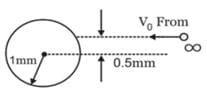Detailed Solution for Test: Electrostatic Potential Energy - Question 1

From conservation of angular momentum,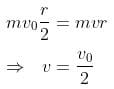from conservation of energy,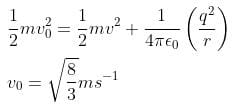Test: Electrostatic Potential Energy - Question 2

### A uniform electric field 100 V/ m is directed vertically downwards. Find the change in potential energy of an electron whose height decreases by 0.5 m.

Detailed Solution for Test: Electrostatic Potential Energy - Question 2

Definition based

Test: Electrostatic Potential Energy - Question 3

### Negative mutual potential energy corresponds to attraction between two charges

Detailed Solution for Test: Electrostatic Potential Energy - Question 3

Definition based

Test: Electrostatic Potential Energy - Question 4

The amount of work done in moving a charge from one point to another along an equipotential line or surface charge is

Detailed Solution for Test: Electrostatic Potential Energy - Question 4

Since Potential difference between two points in equipotential surfaces is zero, the work done between two points in equipotential surface is also zero.

Test: Electrostatic Potential Energy - Question 5

In bringing an electron towards another electron, the electrostatic potential energy of the system

Detailed Solution for Test: Electrostatic Potential Energy - Question 5

Definition based

Test: Electrostatic Potential Energy - Question 6

The potential energy of a system containing only one point charge is

Test: Electrostatic Potential Energy - Question 7

A proton is accelerated from rest through a potential of 500 volts. Its final kinetic energy is

Detailed Solution for Test: Electrostatic Potential Energy - Question 7

K = qV

Test: Electrostatic Potential Energy - Question 8

The electrostatic potential energy between two charges q1 and q2 separated by a distance by r is given by

Detailed Solution for Test: Electrostatic Potential Energy - Question 8

We know that energy required/gained = force x displacement
As F = kqQ / r2 and displacement is r
We get U = kQq /r
Where k = 1/4ϵ

Test: Electrostatic Potential Energy - Question 9

What is the electrostatic potential energy of a system containing two charges 40 nC and 60 nC at a distance of 36 cm?

Detailed Solution for Test: Electrostatic Potential Energy - Question 9

Definition based

*Answer can only contain numeric values
Test: Electrostatic Potential Energy - Question 10

Three point charges Q, +q and +q are placed at the vertices of a right-angled isosceles triangle as shown in the figure. If the net electrostaic energy of the configuration is zero, find the value Q\q is [Take √2 = 1.4]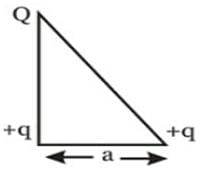Detailed Solution for Test: Electrostatic Potential Energy - Question 10

Net electrostatic energy of the configuration will be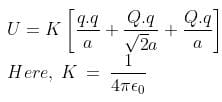Putting U = 0 we get,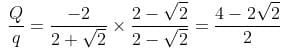-(2-√2) = -0.6

## Physics Class 12

157 videos|425 docs|213 tests
 Use Code STAYHOME200 and get INR 200 additional OFF Use Coupon Code
Information about Test: Electrostatic Potential Energy Page
In this test you can find the Exam questions for Test: Electrostatic Potential Energy solved & explained in the simplest way possible. Besides giving Questions and answers for Test: Electrostatic Potential Energy, EduRev gives you an ample number of Online tests for practice

## Physics Class 12

157 videos|425 docs|213 tests

### How to Prepare for NEET

Read our guide to prepare for NEET which is created by Toppers & the best Teachers